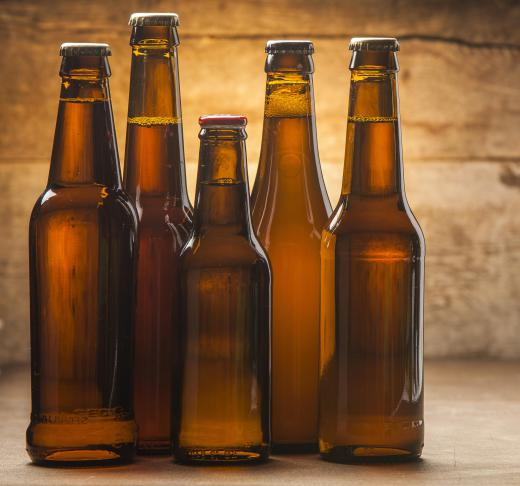Science
Fact Checked

# What Is Charles's Law?

Vincent Summers
Vincent Summers

Charles's law describes the relation between volume and temperature of a gas. The law was credited to French scientist Jacques Charles by chemist Joseph Louis Gay-Lussac, also of France. Simply put, if pressure remains constant, the volume of a gas divided by its temperature is equal to a constant. It can be deduced from the ideal gas equation, PV=nRT, where P is the pressure, V is the volume, n is the number of moles of gas, R is the ideal gas constant and T is the Kelvin temperature. Rewriting the equation, V/T=(nR/P). Since P is constant, V/T=(constant).

Since the ratio equals a constant, V/T=K, a new temperature and volume for the same gas can be written V1/T1=K, leading to V/T= V1/T1. Although this physical relationship is true for an ideal gas, mathematical deviation does occur in the real world, since particle size and interactive forces have not been accounted for. Nevertheless, if temperature is high and pressure is low, particle volume becomes unimportant. Similarly, since pressure is low, particles are far apart. This lessens force interaction between particles, which decreases with the square of the distance, making it insignificant.Charles's law is demonstrated whenever a person opens a bottle or a can of beer or soda.

Although Charles's law is simple, it explains many everyday observations. Yeast in dough gives off tiny bubbles of carbon dioxide gas. Baking that dough expands the bubbles, giving lighter and fluffier pastries. Heating water in a boiler produces expansive steam, which is used to drive steam engines and keep classrooms warm. The gasoline-driven automobile engine burns fuel, producing tremendous heat that expands the combustion gases to drive the pistons that turn the crankshaft and power the vehicle.

Charles's law is demonstrated also whenever a person opens a bottle or a can of beer or soda. Containers of these beverages have pressurized carbon dioxide inside them. If a can or bottle of beverage is cold, opening the top results in very little gas expansion. With the same beverage at a hot temperature, carbon dioxide gas will expand to a much greater degree. This can cause some of the contents to be shot out of the can and onto the consumer.

Another simple application in which Charles's law can shed some light is the filling of a balloon. Volume (V), density (D) and mass (M) obey the relation, D=M/V. Rearranging gives V=M/D. Substituting this into Charles's law V/T=(constant) gives M/DT=(constant). This modification of the law says that if a balloon is filled with gas, and the temperature decreases, the density will increase. If the balloon reaches a point where the outside air has a density similar to that inside the balloon, the balloon will not rise further.

## You might also Like